# Group Target Xbar-s Charts

Group target Xbar-s charts provide information about changes in process averages and the standard deviation across multiple measurement subgroups of similar characteristics that have a common process. Review the following example—an excerpt from Innovative Control Charting1—to get a sense of how a group target Xbar-chart works.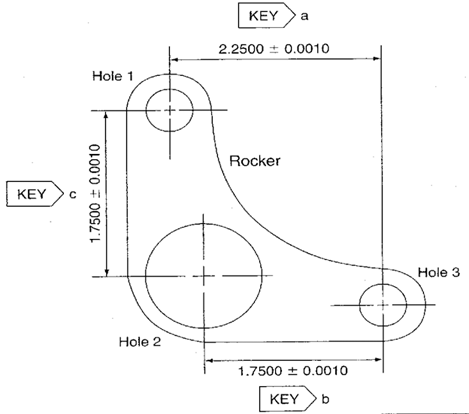Figure 1. Three hole-location measurements from a rocker.

## Case Description

The rocker shown in Figure 1 is machined from an iron casting. There have been complaints from field mechanics that the rockers are not interchangeable and that the holes do not always line up with mating parts. To monitor the uniformity of the hole locations, the operators would like to use a chart at the milling machine to track the variability of the three hole locations.

## Sampling Strategy

Because production volume is very high and all the measurements represent hole locations of different distances created on the same machine, a group target Xbar-s chart is selected. Ten rockers are measured every hour.

## Data Collection Sheet

Table 1. Group target Xbar-s chart data collection sheet for three hole locations on a rocker. MAX and MIN plot points are shown in bold.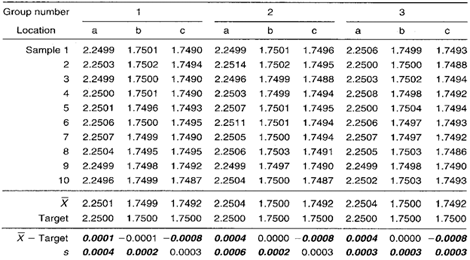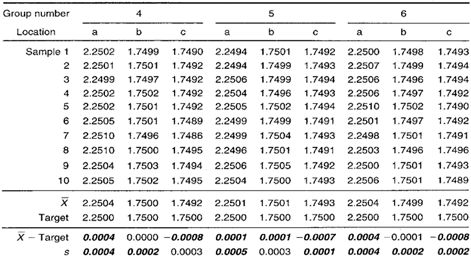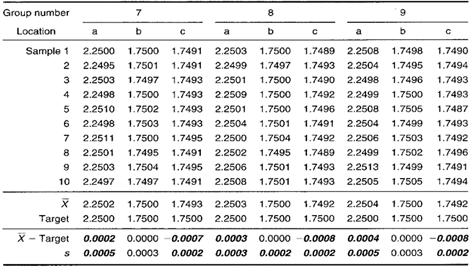## Group Target Xbar-s Chart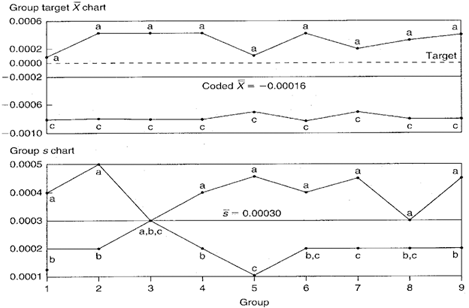Figure 2. Group target Xbar-s chart representing three different hole locations on the same part.

## Chart Interpretation

Group s chart: Location a appears in the MAX position in every group. This indicates that location a has the largest standard deviation. Locations b and c appear randomly in the MIN position, meaning that location b and c’s standard deviation values are both similar to one another and smaller than location a’s.
Note: The centerline on the group s chart is the average of all the sample standard deviation values on the data collection sheet.

Group target Xbar chart: The coded Xbar for location a appears in the MAX. position in every group and its value is always positive. This indicates that the average hole location at location a is consistently higher than the engineering nominal (target) value.

Location c appears in the MIN position in all nine groups and its value is always negative. This means that the average hole location distance at location c is consistently lower than its engineering nominal (target) value.
Note: The centerline on the group target Xbar chart is the average of all the coded Xbar plot points in the data collection sheet.

## Recommendations

• The group target Xbar chart reveals two consistent problems: Location a is always wider than target, and location c is always closer. This type of problem is fixed by changing the location of one or more holes during the job setup. The chart itself does not indicate which hole to relocate. A logical place to begin investigation is with hole 1 because its location affects both key locations a and c.
• Looking at the group s chart, the distance between holes 1 and 3 (hole location a) varies more than the other hole relationships. This also means there is excess variation in the horizontal axis. Operators should verify this assumption with process engineers and remedy the problem..

## Estimating the Process Average

If all of the locations on the group target Xbar chart were behaving randomly, a single estimate of the process average could be used to estimate the process average for all locations. However in this case, the group target Xbar chart does not exhibit random behavior.

Given nonrandom patterns on a group target Xbar chart, estimates of the process average should be calculated separately for each characteristic or location. This is illustrated in Calculation 1 using data from hole location a.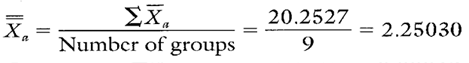Calculation 1. Estimate of the process average for hole location a.

## Estimating Sigma

Estimates of sigma are also calculated separately for each characteristic or location on the group target chart. Continuing with hole location a, see Calculations 2 and 3.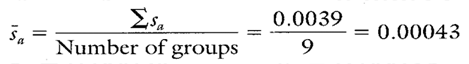Calculation 2. Calculation of s for use in estimating the process standard deviation for hole location a.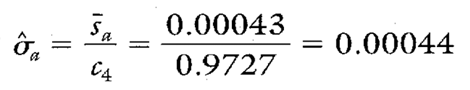Calculation 3. Estimate of the process standard deviation for hole location a.
Note: To ensure reliable estimates, the number of groups should be at least 20. In this example, the number of groups is only nine. Therefore, the estimates here and in Table 2 are for illustration purposes only.

## Calculating Process Capability and Performance Ratios

The Cp and Cpk calculations for hole location a are shown in Calculations 4, 5, and 6.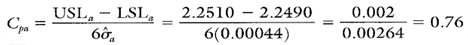Calculation 4. Cp calculation for hole location a.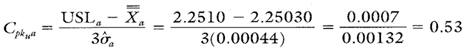Calculation 5. Cpk upper calculation for hole location a.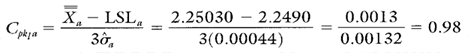Calculation 6. Cpk lower calculation for hole location a.

## Group Target Xbar-s Chart Advantages

• Simultaneously illustrates the variation of multiple product or process characteristics.
• Similar characteristics with different averages can be analyzed on the same chart.
• Separates variation due to changes in the average from variation due to changes in the standard deviation.
• Multiple characteristics can be tracked on one chart.

## Group Target Xbar-s Chart Disadvantages

• No visibility of the characteristics that fall between the MAX and MIN plot points.
• The use of negative numbers can be confusing.
• Cannot detect certain nonrandom conditions because the group target charts described here have no control limits.

The process capability and performance values for hole locations b and c are shown in Table 2.

Table 2. Summary statistics and process capability and performance ratios for hole locations b and c.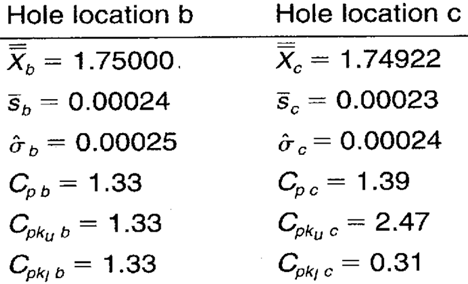When you use SPC software from InfinityQS, consuming the information provided by group target Xbar-charts becomes faster and easier than ever. See how this type of analysis is surfaced in InfinityQS solutions.

FOOTNOTE:
1 Wise, Stephen A. and Douglas C. Fair. Innovative Control Charting: Practical SPC Solutions for Today’s Manufacturing Environment. Milwaukee, WI: ASQ Quality Press.

### Take the first step from quality to excellence

Take the Next Steps